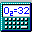##Element Subtraction Using the > Symbol

Element Subtraction was added to allow one to quickly find the mass or empirical formula of a compound that has lost one or more elements due to a reaction or a neutral loss in mass spectrometry. For example:

C6H5Cl>HCl means C6H5Cl minus HCl, having a mass of 76.0959 Da (average mass), equivalent to C6H4

The elements to the right of the > symbol must all be present in the elements to the left of the > symbol, otherwise an error will occur. Furthermore, the number of atoms of a given element listed to the right of the > symbol cannot be greater than the number of atoms of the element to the left of the > symbol. Thus, the following formulas will result in an error:

 C6H5Cl>HBr since Br is not present in C6H5Cl C6H5Cl>HCl2 since only one Cl atom is present in C6H5Cl

If you include multiple > symbols in a formula, then the order of operation is right-to-left. For example:

C6H5Cl3>H3Cl2>HCl
will first be converted to
C6H5Cl3>H2Cl
and then to
C6H3Cl2

The mass of C6H3Cl2 is 145.99342 Da (average mass), and consequently this is the mass returned for C6H5Cl3>H3Cl2>HCl
If you choose Convert To Empirical Formula, then the empirical formula returned is C6H3Cl2

Abbreviations may contain formulas having > symbols in them. Thus, if the abbreviation J is defined to be C6H5Cl>HCl (mass 76.0959 Da), then the formula H2J will have a mass of 78.11184 Da. If you choose Expand Abbreviations for the formula H2J, then the formula will be replaced with H2C6H5Cl>HCl. However, if the original formula is J2 and you choose Expand Abbreviations, then we cannot simply replace J2 with (C6H5Cl>HCl)2 since that is an invalid formula. Consequently, J is first converted to its empirical formula, giving (C6H4)2 This same situation applies if J is in the middle of a formula. For example, H2JO2 will be converted to H2C6H4O2 when choosing Expand Abbreviations, or to C6H6O2 when choosing Convert to Empirical Formula.